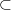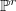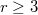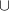﻿

### Postulation of general unions of lines and decorated lines

#### Abstract

A +line A$\subset$$\mathbb {P}^r$ ,$r \geq 3$ , is the scheme A = L$\cup$$v$ with L a line and$v$ a tangent vector of$\mathbb {P}^r$ supported by a point of L, but not tangent to L. Here we prove that a general disjoint union of lines and +lines has the expected Hilbert function.

DOI Code: 10.1285/i15900932v35n1p1

Keywords: postulation; Hilbert function; lines; zero-dimensional schemes

Full Text: PDF